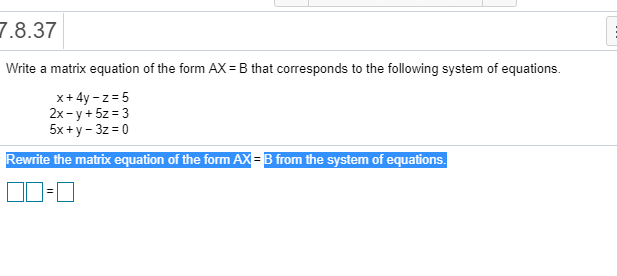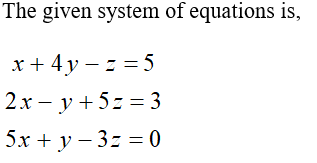# 7.8.37Write a matrix equation of the form AX = B that corresponds to the following system of equations.x+ 4y - z = 52x - y + 5z = 35x +y - 3z = 0Rewrite the matrix equation of the form AX = B from the system of equations.

Question
5 views

How do i solve

picture below ?help_outlineImage Transcriptionclose7.8.37 Write a matrix equation of the form AX = B that corresponds to the following system of equations. x+ 4y - z = 5 2x - y + 5z = 3 5x +y - 3z = 0 Rewrite the matrix equation of the form AX = B from the system of equations. fullscreen
check_circle

Step 1...

### Want to see the full answer?

See Solution

#### Want to see this answer and more?

Solutions are written by subject experts who are available 24/7. Questions are typically answered within 1 hour.*

See Solution
*Response times may vary by subject and question.
Tagged in